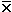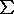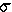Statistical evaluation of the Luria - Delbruck thought experiment

mean(xi) / c
variance2[- xi]2 / c

But in 1943, alternative MOSSOM formula for variance simplifies calculation by mechanical calculating machine.

Both Thought Experiments involve 10 observed
Tonr cells our of 64 total cells

Suppose
probability of induction (a = 10 random events / 64 cells = 15%
observed = 3, 1, 5, & 1 = 10 Tonr colonies
mean
= (3 + 1 + 5 + 1) / 4 = 2.5 Tonr  per culture

variance = [(2.5 - 3)2 + (2.5 - 1)2 + (2.5 - 5)2 + (2.5 - 1)2] / 4 = 2.75
or ( 9 + 1 + 25 + 1) / 4  -  2.52  = 2.75

Induction Hypothesis predicts a Poisson Distribution for rare, random events: variance = mean

Suppose
mutation rate (a) = 2 events / 60 cell divisions = 0.033 mutations / cell / generation
observed = 2, 0, 8, & 0 = 10 Tonr colonies
mean  = (2 + 0 + 8 + 0) / 4 = 2.5 Tonr as before
earlier Tonr mutations leave more offspring (as in Culture 3)
variance [(2.5 - 2)2 + (2.5 - 0)2 + (2.5 - 8)2 + (2.5 - 0)2] / 4 = 10.75
or (4 + 0 + 64 + 0) / 4  2.52 = 10.75
after 5 generations, when the number of Tonr cells has doubled in each culture:
variance' [(5.0 - 4)2 + (5.0 - 0)2 + (5.0 - 16)2 + (5.0 - 0)2] / 4 = 48.00

Mutation Hypothesis predicts variance >> mean, as g increases

All text material ©2017 by Steven M. Carr